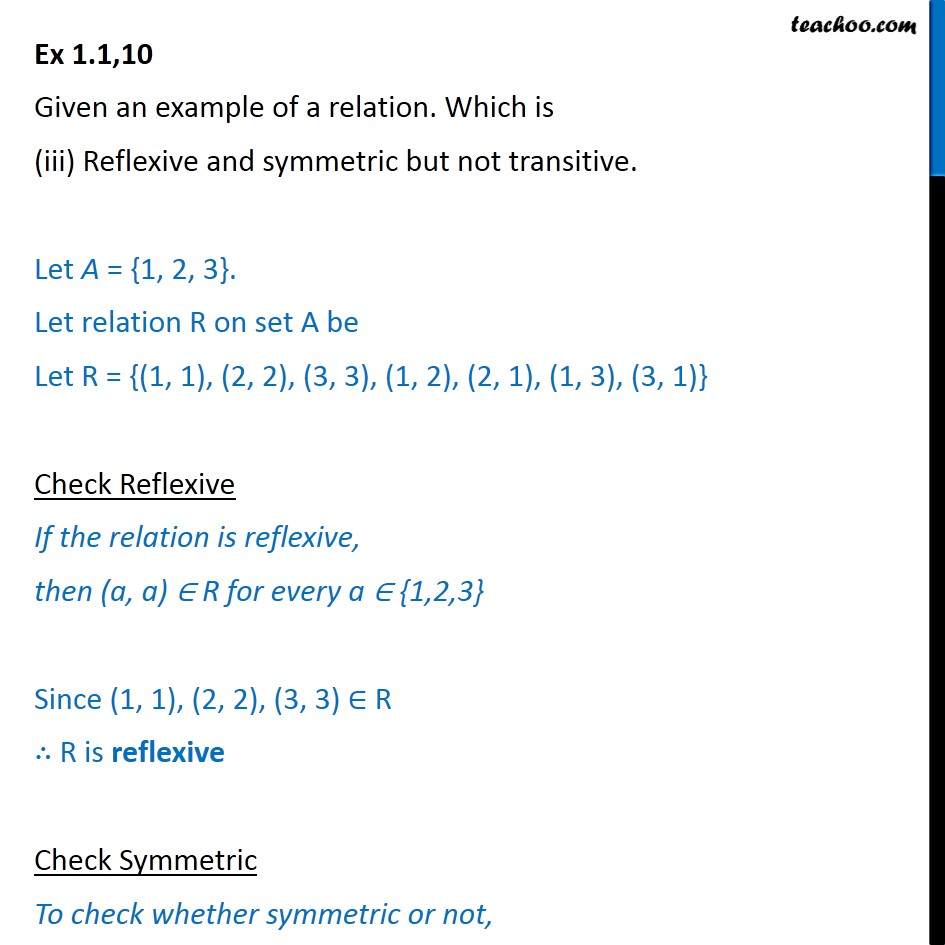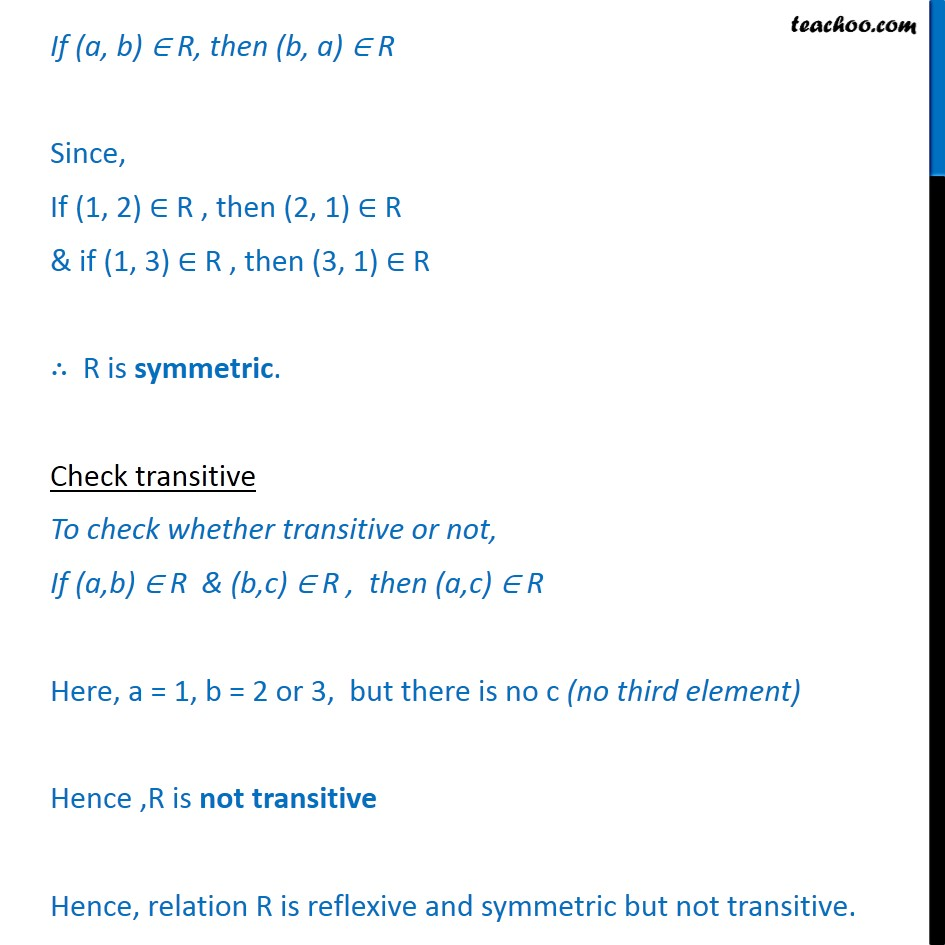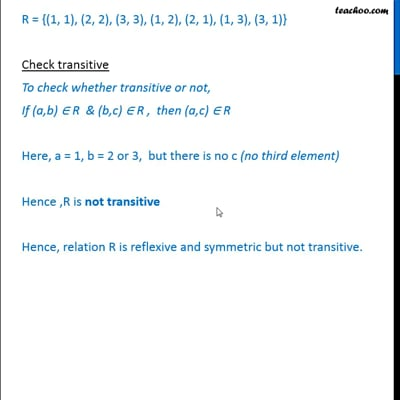Ex 1.1

Chapter 1 Class 12 Relation and Functions
Serial order wiseThis video is only available for Teachoo black users

Maths Crash Course - Live lectures + all videos + Real time Doubt solving!

### Transcript

Ex 1.1,10 Given an example of a relation. Which is (iii) Reflexive and symmetric but not transitive. Let A = {1, 2, 3}. Let relation R on set A be Let R = {(1, 1), (2, 2), (3, 3), (1, 2), (2, 1), (1, 3), (3, 1)} Check Reflexive If the relation is reflexive, then (a, a) R for every a {1,2,3} Since (1, 1), (2, 2), (3, 3) R R is reflexive Check Symmetric To check whether symmetric or not, If (a, b) R, then (b, a) R Since, If (1, 2) R , then (2, 1) R & if (1, 3) R , then (3, 1) R R is symmetric. Check transitive To check whether transitive or not, If (a,b) R & (b,c) R , then (a,c) R Here, a = 1, b = 2 or 3, but there is no c (no third element) Hence ,R is not transitive Hence, relation R is reflexive and symmetric but not transitive.